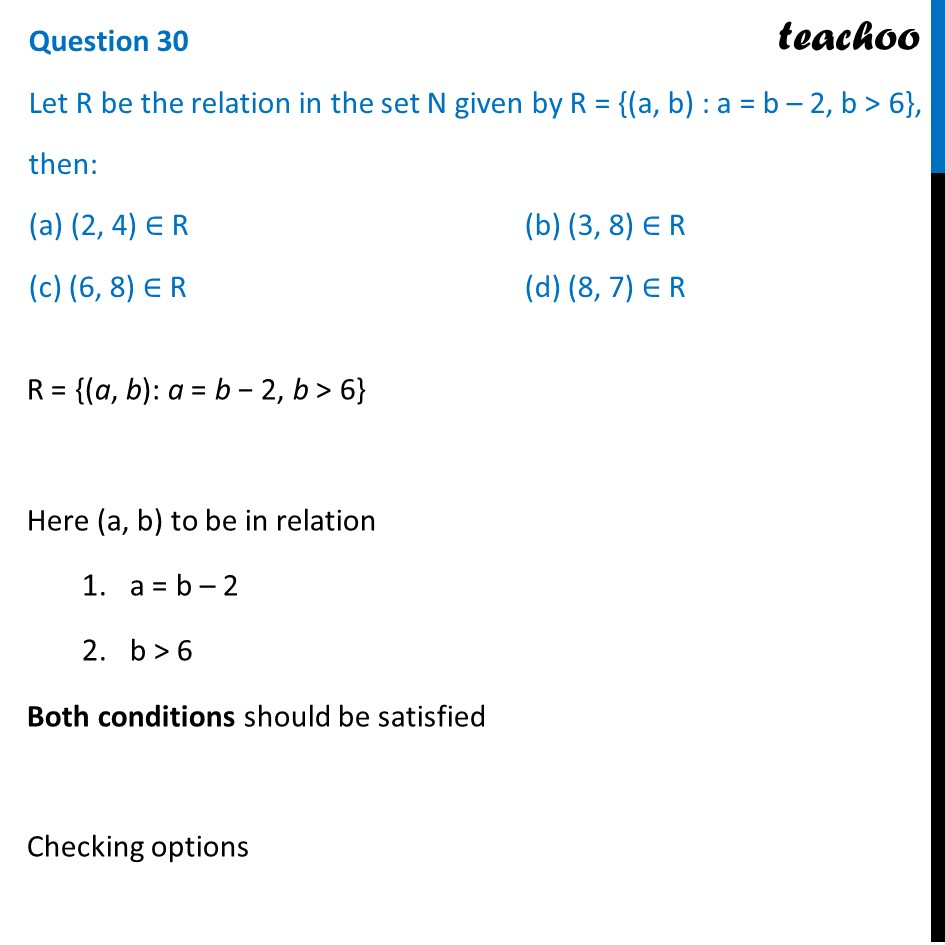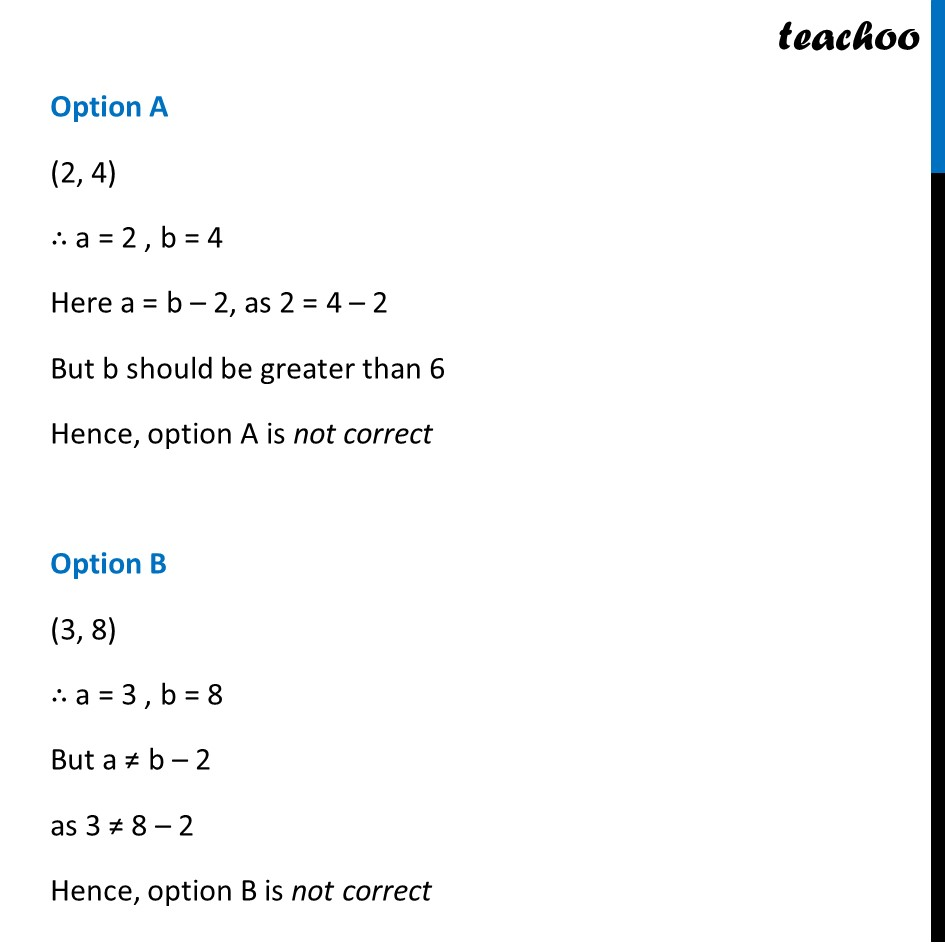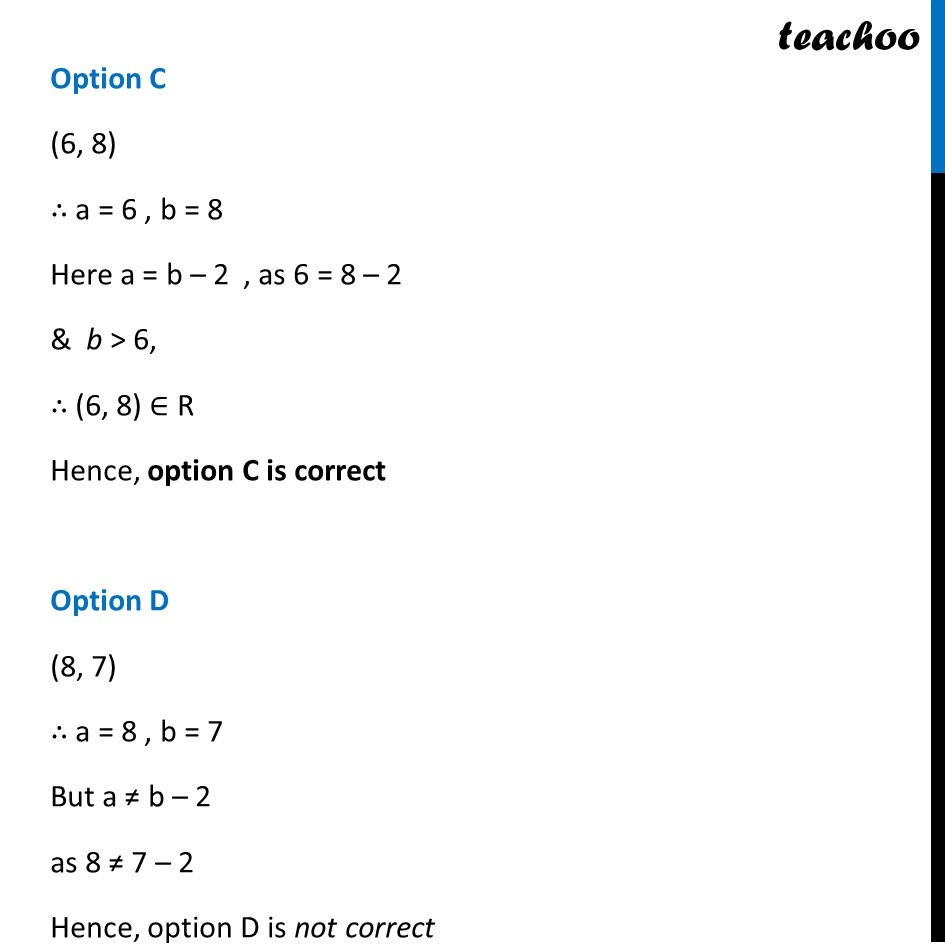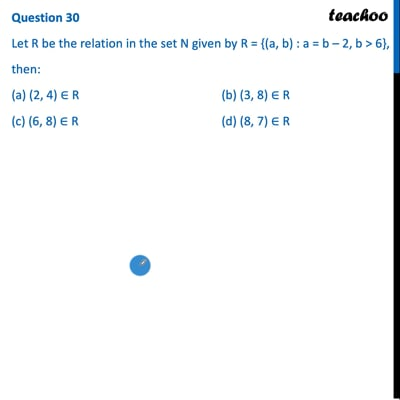CBSE Class 12 Sample Paper for 2022 Boards (MCQ Based - for Term 1)

Class 12
Solutions of Sample Papers and Past Year Papers - for Class 12 Boards

## (c) (6, 8) ∈ R               (d) (8, 7) ∈ R

This question is inspired from Ex 1.1, 16 (MCQ) - Chapter 1 Class 12 - Relation and FunctionsThis video is only available for Teachoo black users

Introducing your new favourite teacher - Teachoo Black, at only ₹83 per month

### Transcript

Question 30 Let R be the relation in the set N given by R = {(a, b) : a = b – 2, b > 6}, then: (a) (2, 4) ∈ R (b) (3, 8) ∈ R (c) (6, 8) ∈ R (d) (8, 7) ∈ R R = {(a, b): a = b − 2, b > 6} Here (a, b) to be in relation a = b – 2 b > 6 Both conditions should be satisfied Checking options Option A (2, 4) ∴ a = 2 , b = 4 Here a = b – 2, as 2 = 4 – 2 But b should be greater than 6 Hence, option A is not correct Option B (3, 8) ∴ a = 3 , b = 8 But a ≠ b – 2 as 3 ≠ 8 – 2 Hence, option B is not correct Option C (6, 8) ∴ a = 6 , b = 8 Here a = b – 2 , as 6 = 8 – 2 & b > 6, ∴ (6, 8) ∈ R Hence, option C is correct Option D (8, 7) ∴ a = 8 , b = 7 But a ≠ b – 2 as 8 ≠ 7 – 2 Hence, option D is not correct